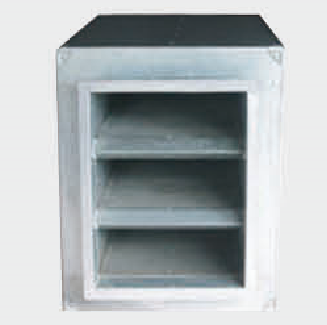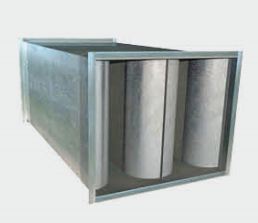3004005006008001000250×160×1000250×200×10001000×400×10001000×500×10001000×630×10001000×800×1000630×320×1000630×400×10001000×320×1000800×400×1000800×800×10001000×1000×10001000×250×10001250×400×10001250×500×10001600×500×10001600×630×10001600×800×1000800×630×10002000×800×1000630×500×1000800×8001250×1000×10001250×630×10001250×800×1000400×200×1000800×320×1000400×400×1000500×200×10001000×1000×5002000×500×1000400×250×1000500×250×1000500×320×1000500×500×300630×630×1000750×750×500320×200×1000500×500×1000800×500×10002000×1250×10002000×630×1000400×320×1000800×250×10001250×320×1000320×250×1000500×400×1000630×250×10001600×1000×10001600×1250×10002000×1000×1000320×320×1000400×400630×200×1000250×250×1000320×160×1000700×700800×400×1500800×800×1500900×9001000×250×15001000×320×15001000×400×15001000×500×15001000×630×15001000×800×15001250×1000×15001250×400×15001250×500×15001250×630×15001250×800×15001600×500×15001600×630×15001600×800×15002000×500×1500600×400×1000630×320×1500630×400×1500630×500×1500630×630×1500800×630×1500900×900×10001000×1000×900250×160800×500800×800×9001000×10001000×4001000×5001000×8002000×2000×10002000×400×10002000×630×15002000×800×15002500×800×1000250×200400×400×900500×500500×500×900800×400900×400×10001000×1000×15001000×800×5001500×600×10001600×400×10002000×1000×15002000×1200×10002000×600×1000200×200×10002500×500×1000400×400×1500500×320×1500500×500×1500600×600×1000630×200×1500800×320×900800×500×15001000×1000×12501000×400×3201000×500×12501000×500×9001000×630×4001000×700×18001200×400×9001200×630×10001250×1250×10001250×1250×5001250×250×10001250×320×15001250×5001250×6301250×8001500×1000×10001500×1200×10001500×1500×10001500×500×9001500×800×10001600×1000×15001600×1250×15001600×600×10001750×1750×10001800×500×9002000×10002000×1250×15002000×1500×10002000×2500×8002000×500×9002250×2250×1000250×160×1500250×200×1500250×250×1500275×275×1000300×300×1000320×160×1500320×200×1500320×250×1500320×320×15003250×32503250×3250×1000350×350×10003750×37503750×3750×1000400×200400×200×1500400×250×1500400×320×1200400×320×1500400×400×12505000×1000×12505000×50005000×5000×1000500×200500×200×1500500×250500×250×1500500×320500×320×1200500×400×1200500×400×1500500×500×1250500×500×500500×500×800600×500×1000600×600×600630×200×1250630×200×900630×250630×250×1500630×300×1000630×300×1250630×300×900630×320630×400630×500630×630×1250630×630×900700×700×900750×750×1000800×250×1500800×300×1000800×300×1250800×320800×320×1500800×400×1250800×800×1250900×400×1500900×600×10001000×3201000×6301250×10001250×12501250×4001600×10001600×12501600×5001600×6301600×8002000×1250630×630800×6301250×250×15001600×400×15002000×400×15002000×5002500×500250×250320×160320×200320×250320×320400×250400×320500×400600×400630×200800×2501000×2501250×3201250×6001750×17502000×8002250×2250300×300750×7501000×1001200×3201200×4001250×1001500×10001500×12501500×4001500×400×10001500×5001500×6301500×8001600×1001600×3201600×320×10001600×4001800×5001800×800×10002000×1002000×1200×15002000×16002000×1600×10002000×20002000×4002000×600×15002000×6302200×5002300×5002400×5002500×20002500×500×15002500×8002500×800×1500275×275500×1000500×800×1000600×400×900640×400D1000D1100D1200D1300D1400D1800D600D700D750D800D900定制

202020192018201713%
2020/11/2713%
2020/11/2713%
2020/11/2713%
2020/11/2713%
2020/11/2713%
2020/11/2713%
2020/11/2713%
2020/11/2713%
2020/11/2713%
2020/11/2713%
2020/11/2713%
2020/11/2713%
2020/11/2713%
2020/11/2713%
2020/11/2713%
2020/11/2713%
2020/11/2713%
2020/11/2713%
2020/11/2713%
2020/11/2713%
2020/11/2713%
2020/11/2713%
2020/11/2713%
2020/11/2713%
2020/11/2713%
2020/11/2713%
2020/11/2713%
2020/11/2713%
2020/11/2713%
2020/11/2713%
2020/11/2713%
2020/11/2713%
2020/11/2713%
2020/11/2713%
2020/11/2713%
2020/11/2713%
2020/11/2713%
2020/11/2713%
2020/11/2713%
2020/11/27

• 1
• 2
• 3
• 4
• 5
• 6
• 62订阅中心广联达大数据